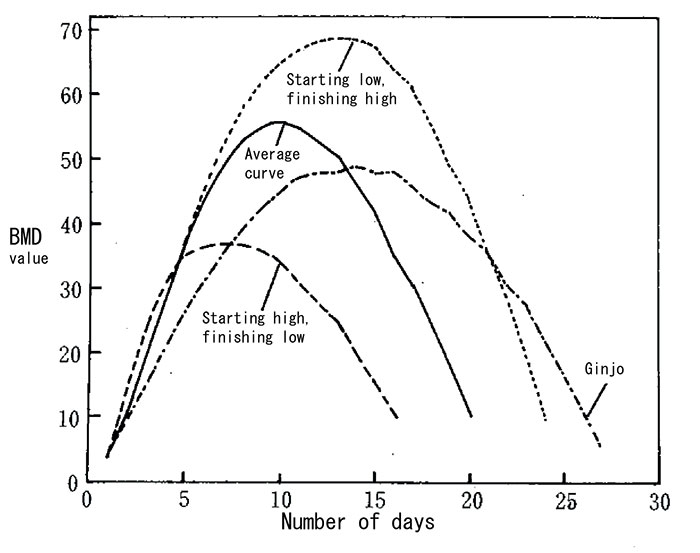# BMD Kyokusen (B kyokusen)

【English】
BMD graph (B graph)

One of the tools for handling moromi is the BMD (or B) kyokusen (curvilinear) graph. The BMD value is calculated as follows

(Number of days after mashing) x ( Baumé value of mash that day)

(Where measurements are taken in SMV units, this figure must be converted to Baumé .)

The graph is plotted with the number of days as the horizontal axis and the BMD value on the vertical, and the result is called the BMD curve. The curve for moromi with a strong-starting fermentation has a low peak; slow starters have a late, high peak. By connecting the highest point on the curve with the point from the following day where it starts to fall and extending that line, it is possible to predict how many days it will take to reach the zero point, and so to judge the timing of pressing.Examples of BMD curves ( Graph from “Seishu Brewing Technology”, p232 )